# Study of rare and search for forbidden decays of charged kaons

Publications, 14 August 2020

The series of studies combines together some results of the NA48/2 kaon rare decays analysis and the results of the NA62 search for the physical phenomena forbidden within the Standard Model. The investigations have been performed in the frameworks of the NA62 project and 1096 theme of the JINR Topical Plan with the defining contribution of scientists of the Veksler and Baldin Laboratory of High Energy Physics JINR. The final results are published in the leading scientific journals. Preliminiry results where presented by the HEP laboratory scientific workers on the international conferences on behalf of the NA48/2 and NA62 collaborations.

The team of authors comprising of E. Goudzovski, V. Kekelidze, D. Madigozhin, M. Misheva, Yu. Potrebenikov, S. Shkarovskiy was awarded for the scientific paper “Study of rare and search for forbidden decays of charged kaons” with the second JINR Prize for 2019 in the section “Experimental research papers”.

### Measurement of the form factors of charged kaon semileptonic decays

Form factors measurement for the semileptonic decays K±→π0l+ν (K±l3, where l = e, µ), together with these decays branching ratio results, are contributing to the precise determination of the CKM matrix element absolute value |Vus|. In the frameworks of the NA48/2 experiment, JINR group has performed a new measurement of the vector f+ and scalar f0 form factors for the Kе3 and Kμ3 decays using three most used form factor parameterizations, as well as the form factors combined measurement for Kl3.

The Dalitz plots for the selected events are shown in figure 1. These plots after background correction have been used to extract the form factor parameters by means of fits. The full statistics of selected events is 4.4∙106 for Ke3 and 2.3∙106 for Kμ3. The largest background contributions comes from the kaon decays to π±π0 and π±π0π0 (0.6∙10-3 and 2.5∙10-3 for Ke3 and Kμ3, respectively). In order to demonstrate the fits quality, figure 2 shows the background corrected experimental distributions on the pion and lepton energies in the kaon center of mass together with the fit results obtained using the Taylor expansion for the form factors description.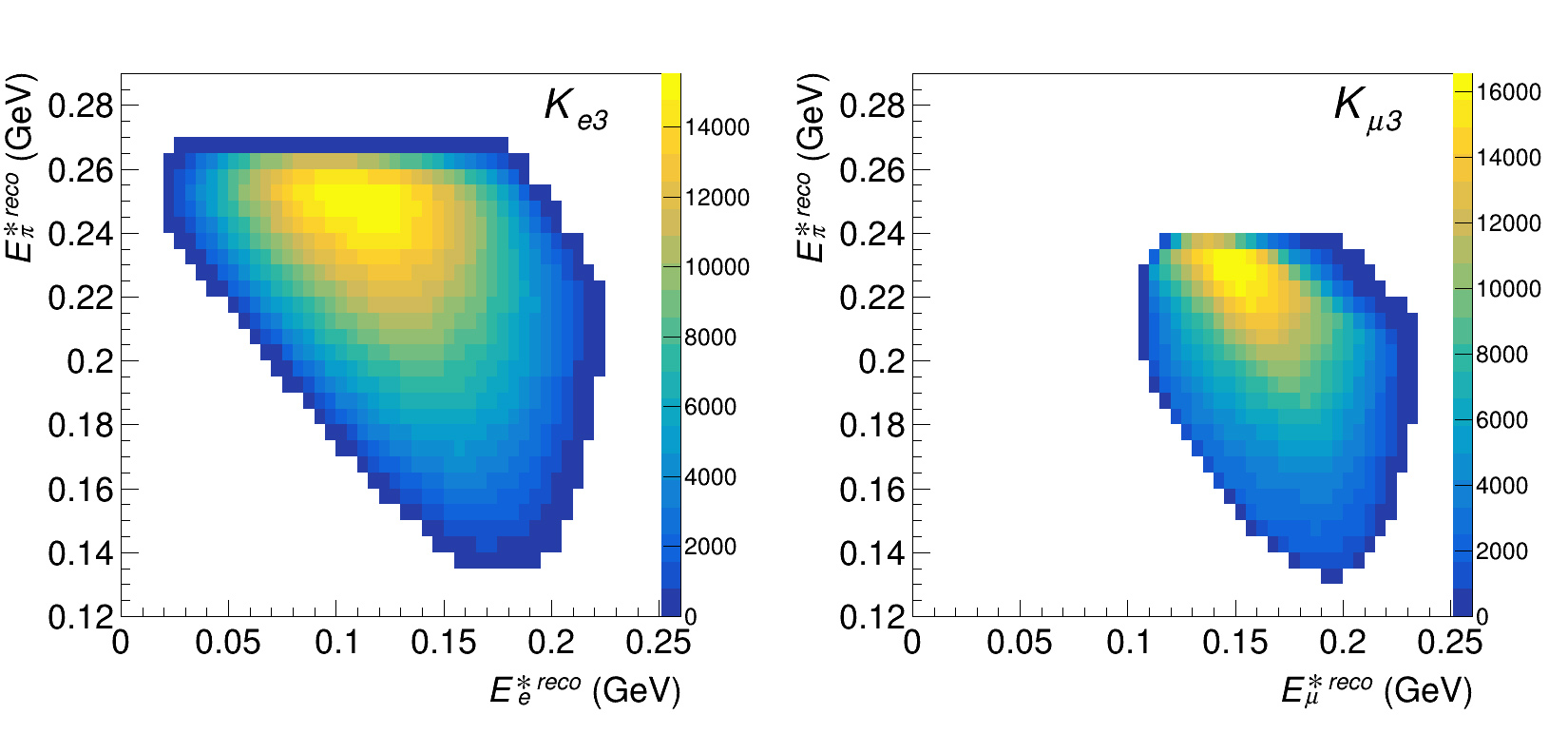Figure 1. Measured pion and lepton energy distributions in the kaon center of mass for selected K±l3 decays (Dalitz plotsFigure 2. Reconstructed experimental lepton and pion energy distributions after background subtraction and fit results obtained using the Tailor expansion for the form factors approximation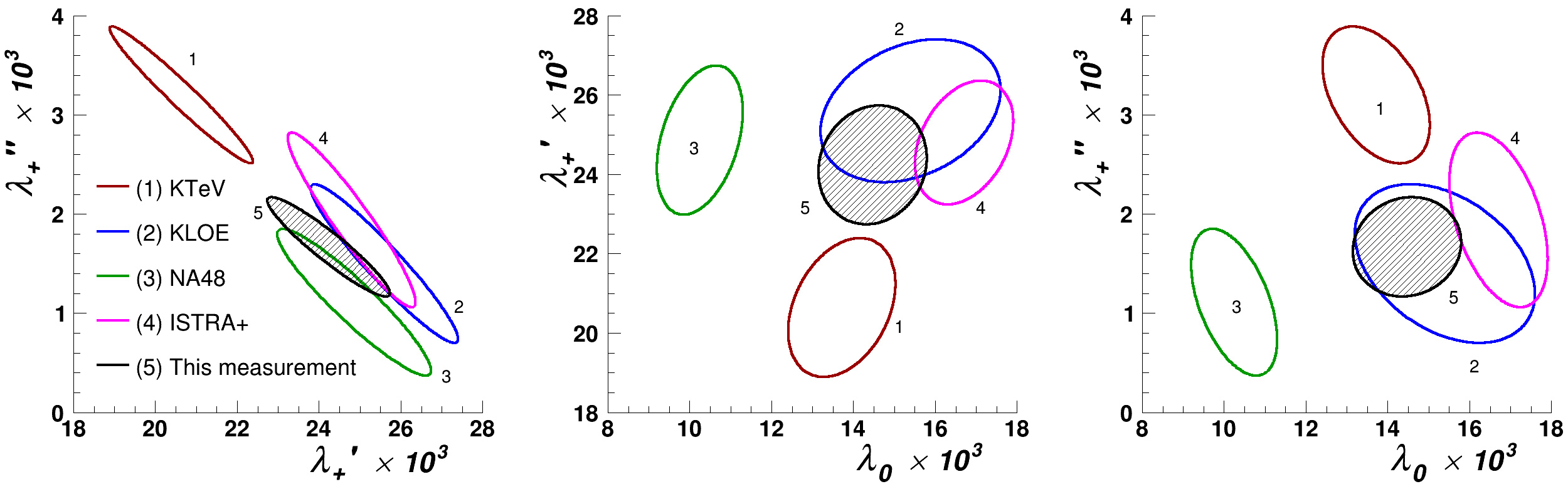Figure 3. One sigma (39.4% CL) contours for the obtained parameters of the Taylor expansion. Results of the combined Ke3 and Kμ3 analysis are shown

The results of the NA48/2 combined measurement for Ke3 and Kμ3, together with the earlier results from KTeV, KLOE, NA48, and ISTRA+ experiments, are shown in figure 3. The present results are in agreement with the previously recognized measurements. At the moment, NA48/2 provides the most precise combined measurement results.

### First observation and study of the K±→π±π0e+e– decay

On the basis of data collected by NA48/2 experiment in 2003-2004, 4919 K±→π±π0e+e candidates have been selected, and the first measurement of the corresponding branching ratio has been performed: BR(K±→π±π0e+e) = (4.237 ± 0.063stat ± 0.033syst ± 0.126ext)∙10-6.

The radiative decay K±→π±π0e+e, never observed so far, proceeds through virtual photon exchange followed by internal conversion into an electron-positron pair. The decay is interesting as a test of low energy QCD models, such as chiral perturbative theory (ChPT), that is an efficient field theory describing strong interactions at the momentum transfer below 1 GeV.

The decay K±→π±π0D has been chosen as a normalization process for the K±→π±π0e+e branching ratio measurement. 16.3∙106 normalization candidates have been selected. The background contamination for π±π0e+e decay has been estimated to be 4.9%, while the background for the normalization channel π±π0D is 0.11%. The signal reconstructed mass distributions for π0→γγ and K±→π±π0e+e are shown in the figure 4. Figure 5 shows the respective mass distributions of the π0→γe+e and K±→π±π0D combinations for the normalization mode.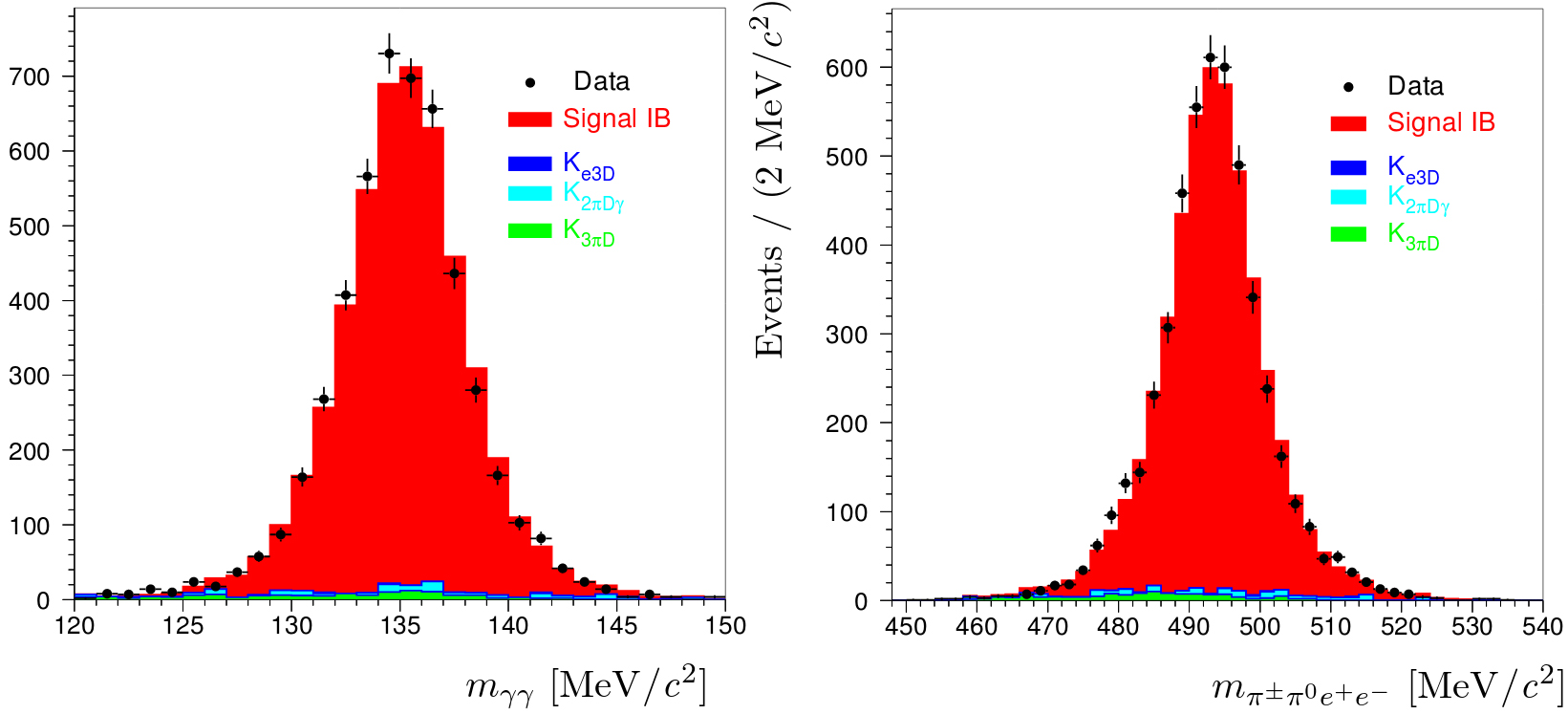Figure 4. K±→π±π0e+e signal candidates. Left: π0→γγ reconstructed mass. Right: K±→π±π0e+e mass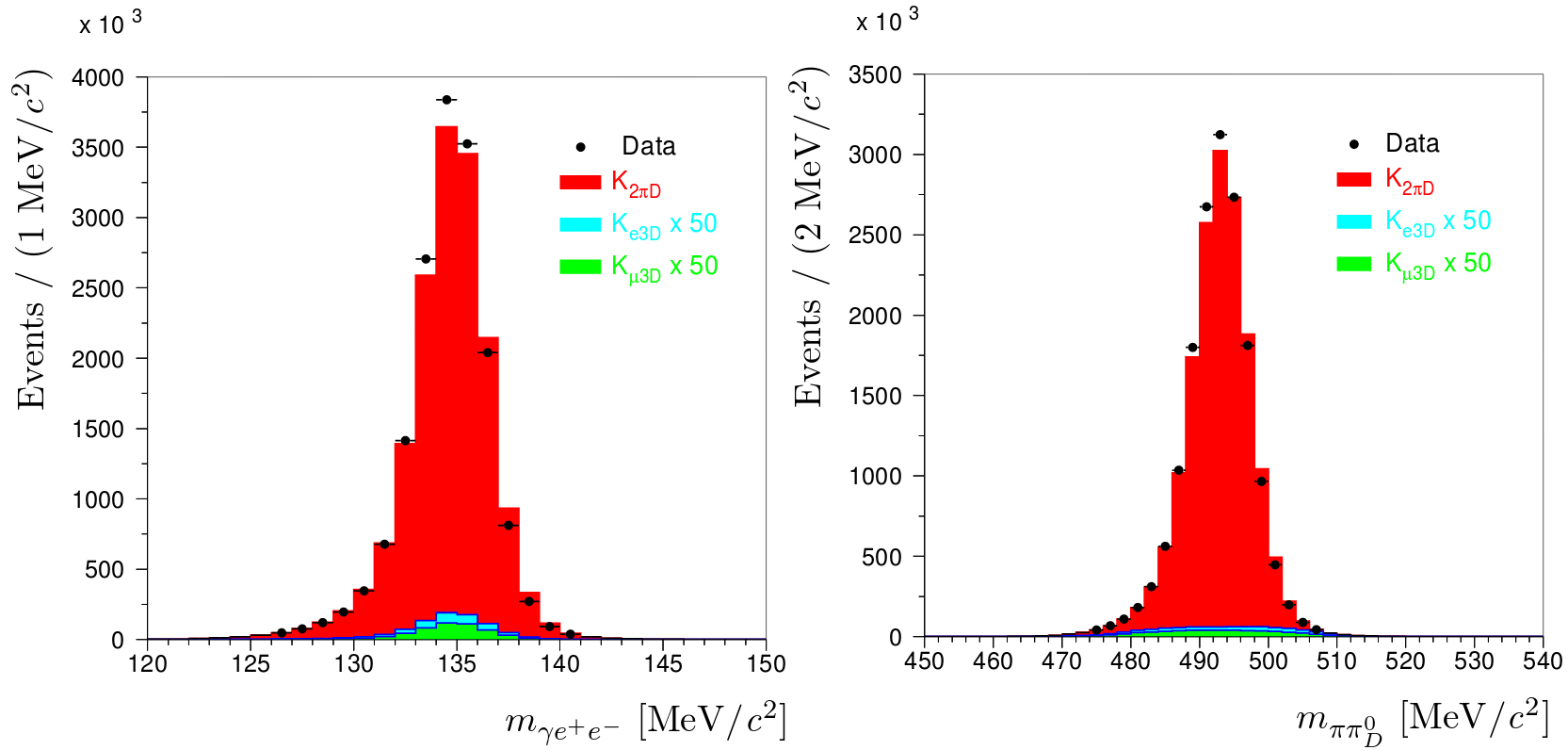Figure 5. Normalization event K±→π±π0D candidates. Left: reconstructed π0→γe+e mass. Right: reconstructed K±→π±π0D mass

The result of the branching ratio measurement is BR(K±→π±π0e+e) = (4.237 ± 0.063stat ± 0.033syst ± 0.126ext)∙10-6. Systematic error is caused predominantly by the radiative effects uncertainty, while the external error (ext) is defined by the uncertainty of the π0D decay branching ratio.

### Search for the double neutrinoless kaon decays K+→π–e+e+ and K+→π–μ+μ+

A search for the lepton number violating double neutrinoless kaon decays K+→πe+e+ and K+→πμ+μ+ has been performed with the best sensitivity using a data sample collected by the NA62 experiment at CERN. In the frameworks of the NA62 data collection system, the trigger for kaon decays into lepton pairs has been proposed and designed by the group under coordination of the JINR VBLHEP scientist. The physical analysis also has been performed by the HEP laboratory scientific worker, who is a coordinator of the NA62 working group on the rare and forbidden decay studies.

In the frameworks of the Standard Model, all neutrinos have zero masses, but the discovery of neutrino oscillations means that in reality neutrino masses deviate from 0. This fact motivates a search for the lepton number violating processes, as their observation would lead to the discovery of the neutrino Majorana nature.

Fig. 6 shows the reconstructed mass distributions of the systems K+→πe+e+ and K+→πμ+μ+ for the selected events of experimental data and for the MC simulated background sources.

The expected background levels in the signal regions are 0,16 ± 0,03 and 0,91 ± 0,41 events, while the amounts of the actually observed data events are 0 и 1, respectively. This fact allows us to set the upper limits for the partial widths of these decays 2,2 ∙ 10-10 и 4,2 ∙ 10-11 at 90% CL, respectively. The obtained results improve the world data related to the possible lepton number violation. The analysis of 2018 data will make the result sensitivity better.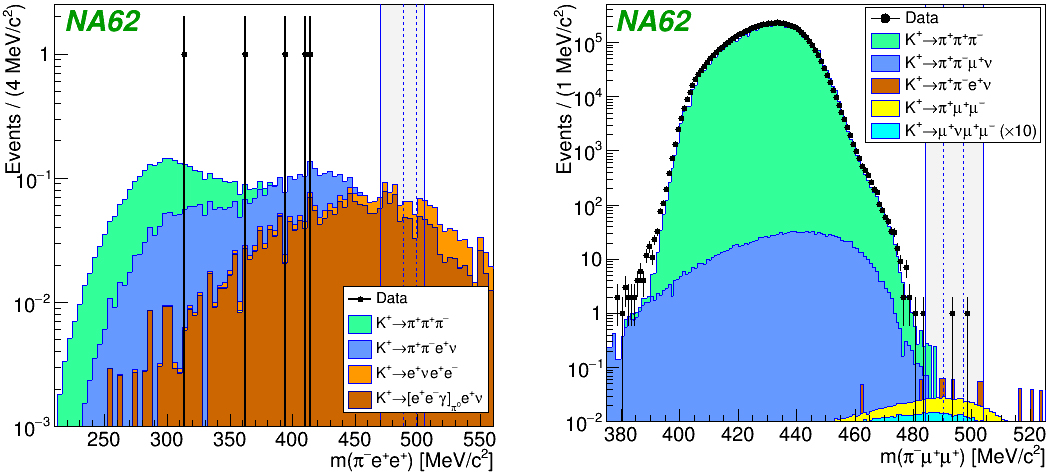Figure 6. Reconstructed mass distributions for K+→πe+e+ (left) and K+→πμ+μ+ (right). The vertical lines show the expected signal region near the kaon mass

### Search for production of heavy neutral lepton in K+ decays

A search for heavy neutral lepton production has been performed in K+ decays to electron or muon and neutral lepton with the mass in the range 170-448 MeV/с2, using a data sample collected with a minimum bias trigger by the NA62 experiment at CERN in 2015.

Some Standard Model extensions predict new particles, such as heavy neutral leptons (HNL, also denoted below as N) or heavy neutrino, that could be produced in kaon decays. A mixing between HNL and the Standard Model ordinary neutrino may lead to the HNL production in some meson decays, including K+→l+N (l = e, μ). The decay branching ratio in this case is defined by the HNL mass mN and by the mixing parameter |Ul4|2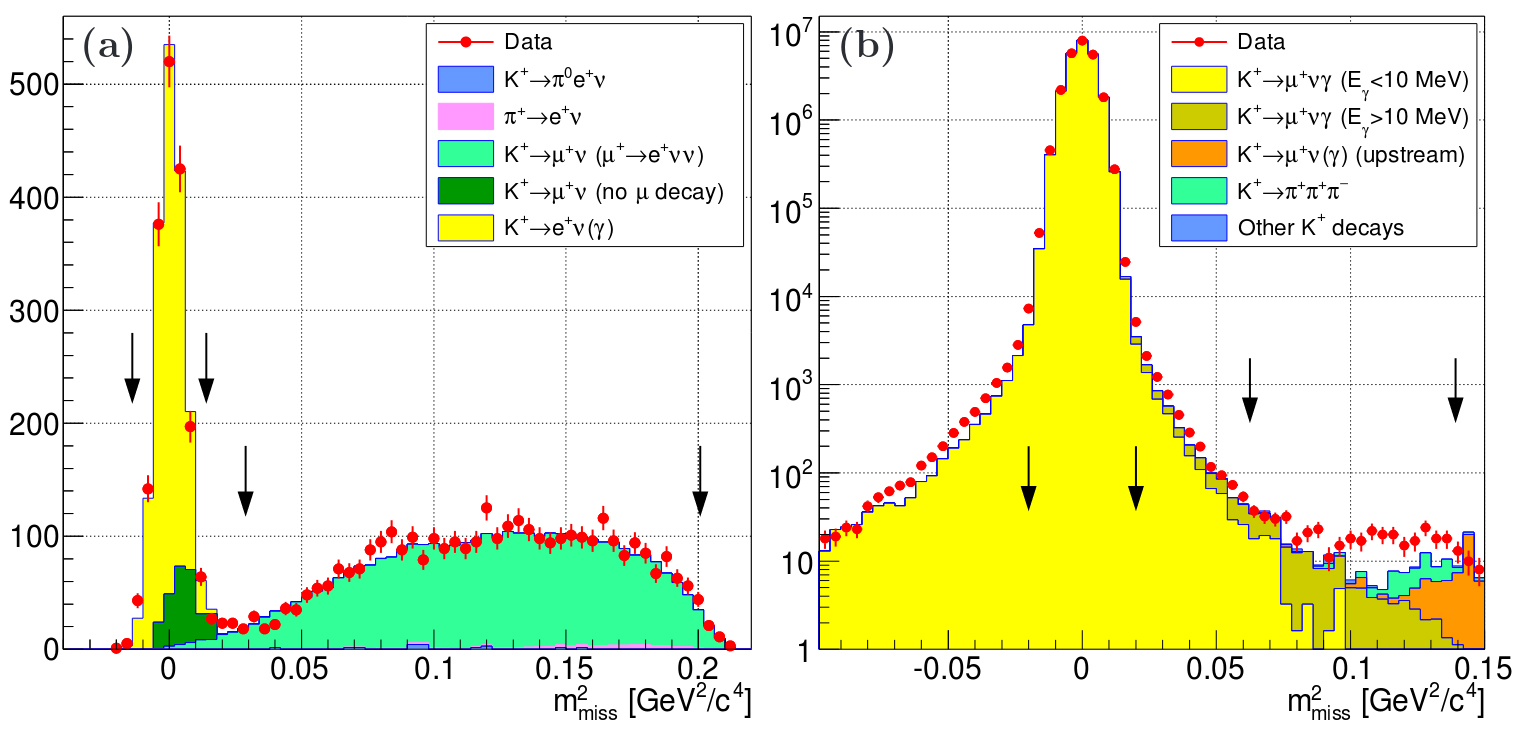Figure 7. Squared missing mass distributions for the experimental data and for MC simulation results. (a) – events with e+; (b) – events with μ+. Vertical arrows show the expected signal regions for the ordinary neutrino (near zero) and for HNL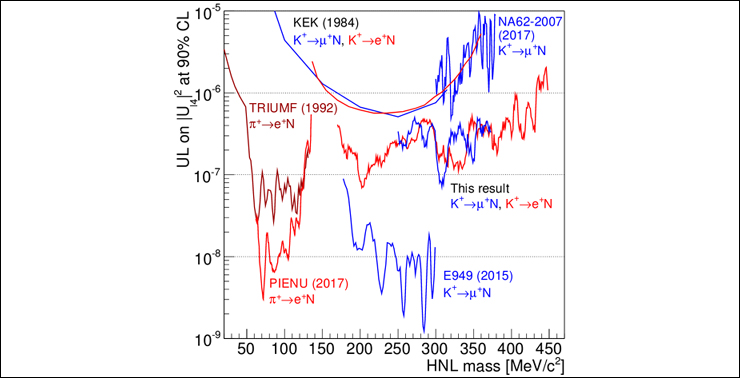Figure 8. Upper limits for |Ul4|2 at 90% CL for different assumed HNL masses

Figure 7 shows the distributions of the missing mass squared for selected events of experimental data and MC simulation results. The search for HNL signal have been performed in the regions shown on the figure by means of the black arrows: 0.03–0.20 GeV2/c4 for e+ and 0.06–0.14 GeV2/c4 for μ+. The search for K+→l+N decay consisted of the attempt to detect a peak over the background in the assumed HNL mass interval.

The obtained upper limits for |Ul4|2 at 90% CL are shown in the figure 8 together with the results of earlier studies of HNL production in π+ decays (TRIUMF, PIENU) and K+ decays (KEK, E949, NA62-2007). The new result improves on the previous limits for |Ue4|2 in the mass range over 300 MeV/с2.